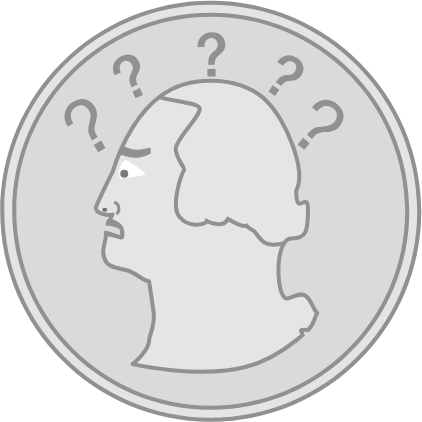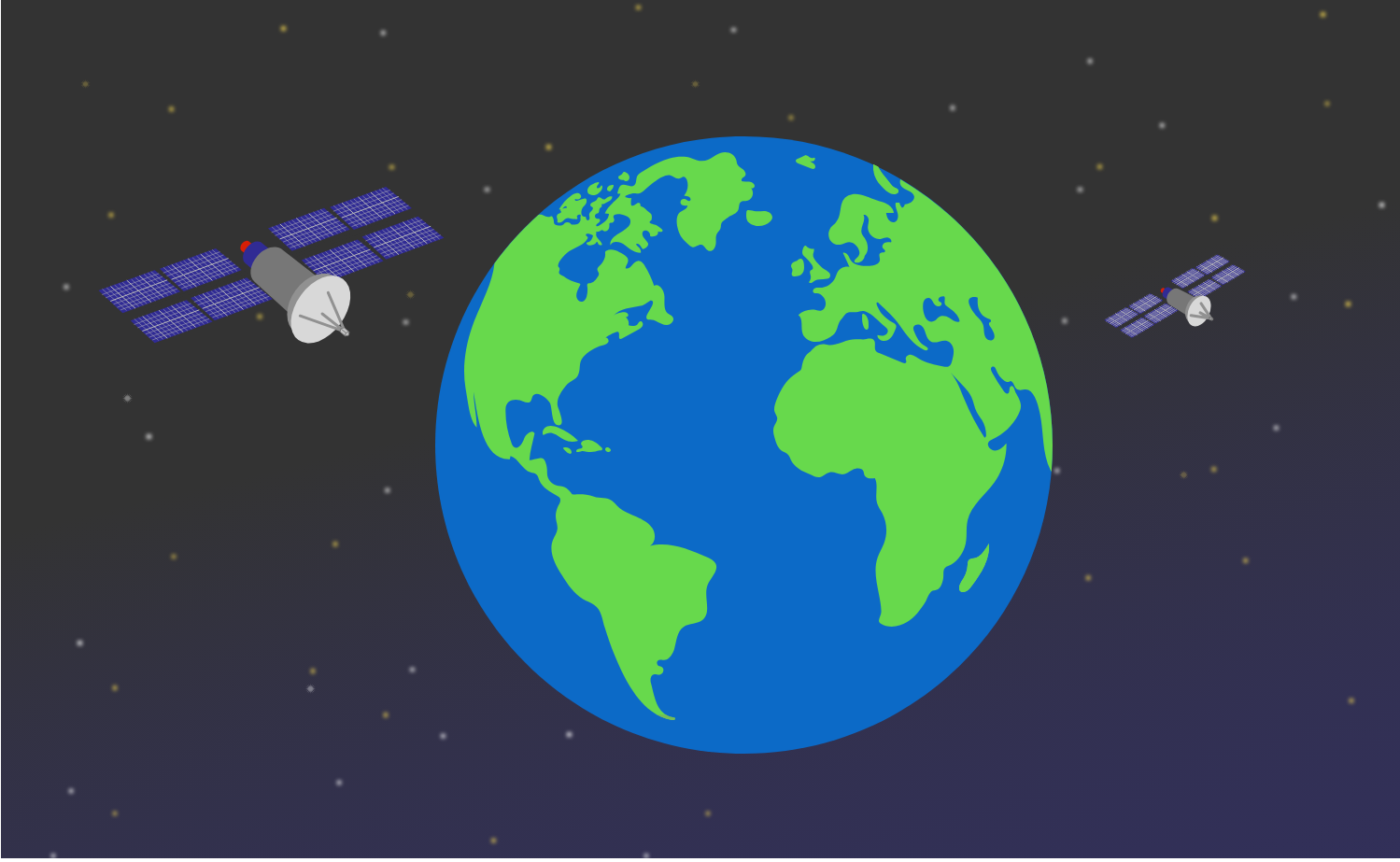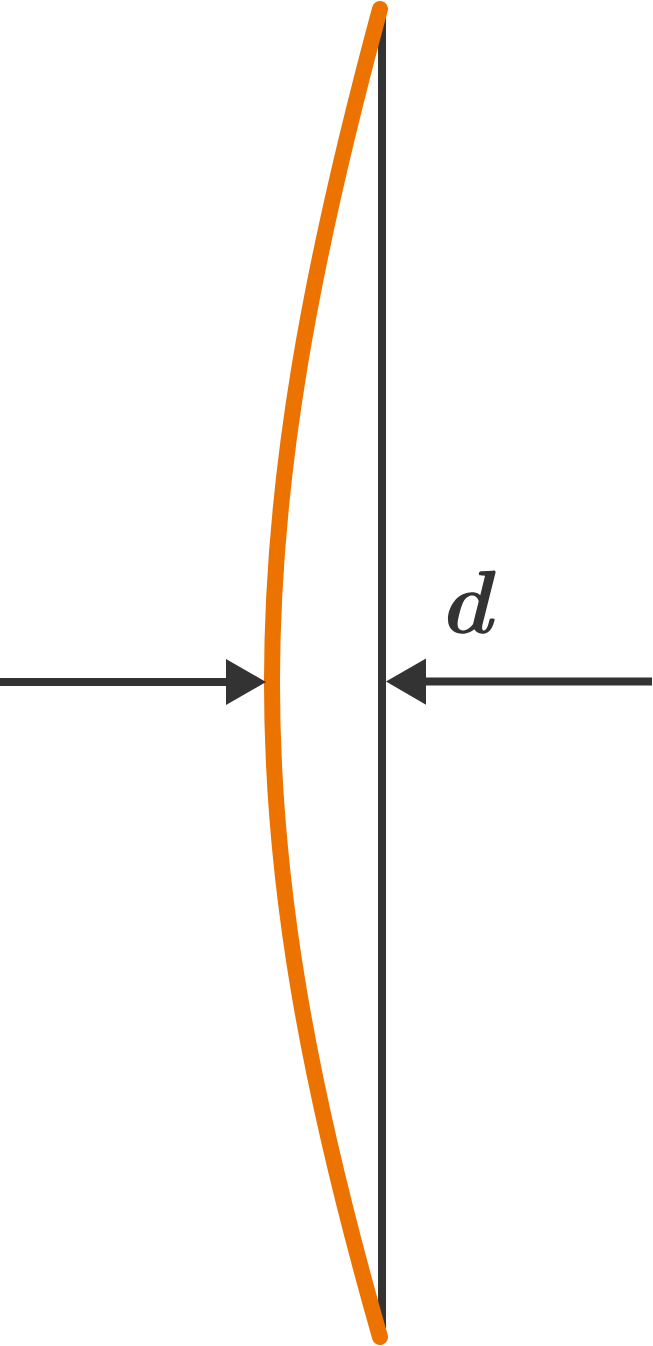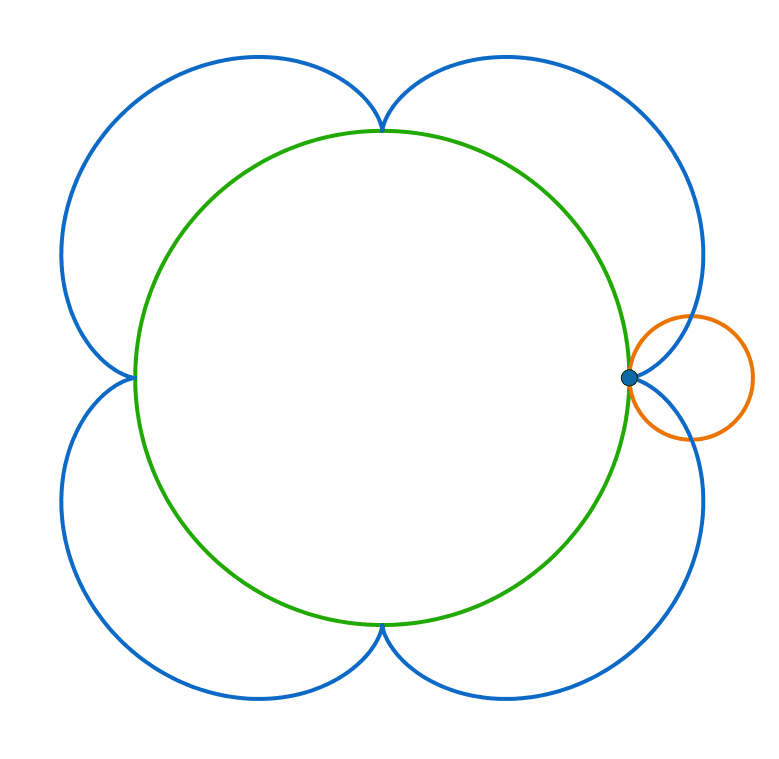# Problems of the Week

Contribute a problem

# 2018-10-29 IntermediateYou have a coin that is biased, although you don’t know its probability of flipping heads or tails.

Using only this coin, can you design a repeatable process that is effectively the same as a fair coin flip?

Assume that the flip outcomes (heads and tails) each have constant non-zero probabilities. The process you design can be as long as it needs to be.

It can be shown that, for all positive integers $n$,

• $n^3 -n$ is divisible by 6 and
• $n^5 - n$ is divisible by 30.

Find the largest positive integer $M$ such that the following is true:

$n^7 - n$ is divisible by $M$ for all positive integers $n.$

Two communication satellites are in Earth's orbit at altitudes of 96 miles and 137 miles, respectively.

If a signal must be sent between them in a straight line without Earth obstructing it, what is the maximum distance (in miles) that the satellites can be from each other?

Round your answer to the nearest hundred. Assume that Earth is a perfect sphere and that its radius is 4000 miles.A bow is made of a straight piece of wood of length $s$ with uniform cross-section. The string is tightened and the bow is slightly bent, as shown.

The distance between the middle point of the bow and the string is $d,$ which is much less than the length of the bow $($i.e. $d\ll s).$

What happens to the tension force in the string if the string is shortened so that the distance $d$ is doubled?

The two circles in the animation have radii $1\text{ cm}$ and $4\text{ cm},$ respectively.

How long is the blue curve traced by the blue point on the smaller circle (in centimeters)?×